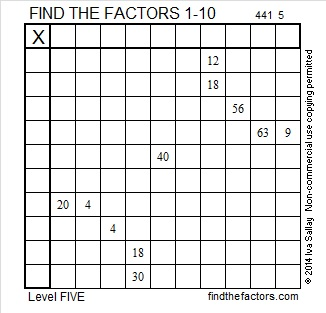265 and Level 5

• 265 is a composite number.
• Prime factorization: 265 = 5 x 53
• The exponents in the prime factorization are 1 and 1. Adding one to each and multiplying we get (1 + 1)(1 + 1) = 2 x 2 = 4. Therefore 265 has 4 factors.
• Factors of 265: 1, 5, 53, 265
• Factor pairs: 265 = 1 x 265 or 5 x 53
• 265 has no square factors that allow its square root to be simplified. √265 ≈ 16.279Here is today’s puzzle:Print the puzzles or type the factors on this excel file: 10 Factors 2014-10-13